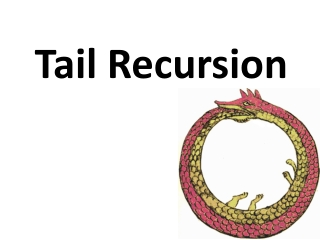DownloadDownload PresentationTail Recursion

# Tail Recursion

Télécharger la présentation## Tail Recursion

- - - - - - - - - - - - - - - - - - - - - - - - - - - E N D - - - - - - - - - - - - - - - - - - - - - - - - - - -
##### Presentation Transcript

1. Tail Recursion

2. Problems with Recursion • Recursion is generally favored over iteration in Scheme and many other languages It’s elegant, minimal, can be implemented with regular functions and easier to analyze formally • It can also be less efficient more functional calls and stack operations (context saving and restoration) • Running out of stack space leads to failure deep recursion

3. Tail recursion is iteration • Tail recursion is a pattern of use that can be compiled or interpreted as iteration, avoiding the inefficiencies • A tail recursive function is one where every recursive call is the last thing done by the function before returning and thus produces the function’s value

4. Scheme’s top level loop • Consider a simplified version of the REPL (define (repl) (printf “> “) (print (eval (read))) (repl)) • This is an easy case: with no parameters there is not much context

5. Scheme’s top level loop 2 • Consider a fancier REPL (define (repl) (repl1 0)) (define (repl1 n) (printf “~s> “ n) (print (eval (read))) (repl1 (add1 n))) • This is only slightly harder: just modify the local variable n and start at the top

6. Scheme’s top level loop 3 • There might be more than one tail recursive call (define (repl1 n) (printf “~s> “ n) (print (eval (read))) (if (= n 9) (repl1 0) (repl1 (add1 n)))) • What’s important is that there’s nothing more to do in the function after the recursive calls

7. Two skills • Distinguishing a trail recursive call from

8. Naïve recursive factorial (define (fact1 n) ;; naive recursive factorial (if (< n 1) 1 (* n (fact1 (sub1 n)))))

9. Tail recursive factorial (define (fact2 n) ; rewrite to just call the tail-recursive ; factorial with the appropriate initial values (fact2-helper n 1)) (define (fact2-helper n accumulator); tail recursive factorial calls itself as; last thing to be done (if (< n 1) accumulator (fact2-helper (sub1 n) (* accumulator n))))

10. |(fact1 6) | (fact1 5) | |(fact1 4) | | (fact1 3) | | |(fact1 2) | | | (fact1 1) | | | |(fact1 0) | | | |1 | | | 1 | | |2 | | 6 | |24 | 120 |720 720 Trace shows what’sgoing on > (require (lib "trace.ss")) > (load "fact.ss") > (trace fact1) > (fact1 6)

11. fact2 • Interpreter & compiler note the last expression to be evaled & returned in fact2-helper is a recursive call • Instead of pushing state on the sack, it reassigns the local variables and jumps to beginning of the procedure • Thus, the recursion is automatically transformed into iteration > (trace fact2 fact2-helper) > (fact2 6) |(fact2 6) |(fact2-helper 6 1) |(fact2-helper 5 6) |(fact2-helper 4 30) |(fact2-helper 3 120) |(fact2-helper 2 360) |(fact2-helper 1 720) |(fact2-helper 0 720) |720 720

12. Reverse a list • This version works, but has two problems (define (rev1 list) ; returns the reverse a list (if (null? list) empty (append (rev1 (rest list)) (list (first list)))))) • It is not tail recursive • It creates needless temporary lists

13. A better reverse (define (rev2 list) (rev2.1 list empty)) (define (rev2.1 list reversed) (if (null? list) reversed (rev2.1 (rest list) (cons (first list) reversed))))

14. rev1 and rev2 > (load "reverse.ss") > (trace rev1 rev2 rev2.1) > (rev1 '(a b c)) |(rev1 (a b c)) | (rev1 (b c)) | |(rev1 (c)) | | (rev1 ()) | | () | |(c) | (c b) |(c b a) (c b a) > (rev2 '(a b c)) |(rev2 (a b c)) |(rev2.1 (a b c) ()) |(rev2.1 (b c) (a)) |(rev2.1 (c) (b a)) |(rev2.1 () (c b a)) |(c b a) (c b a) >

15. The other problem • Append copies the top level list structure of it’s first argument. • (append ‘(1 2 3) ‘(4 5 6)) creates a copy of the list (1 2 3) and changes the last cdr pointer to point to the list (4 5 6) • In reverse, each time we add a new element to the end of the list, we are (re-)copying the list.

16. Append (two args only) (define (append list1 list2) (if (null? list1) list2 (cons (first list1) (append (rest list1) list2))))

17. Why does this matter? • The repeated rebuilding of the reversed list is needless work • It uses up memory and adds to the cost of garbage collection (GC) • GC adds a significant overhead to the cost of any system that uses it • Experienced Lisp and Scheme programmers avoid algorithms that needlessly consume cons cells

18. Fibonacci (define (fib n) ;; naive recurseive fibonacci function (if (< n 3) 1 (+ (fib (- n 1)) (fib (- n 2))))) Run time for fib(n) ≅ O(2n)

19. Fibonacci (define (fib2 n) (if (< n 3) 1 (fib-tr 3 n 1 1))) (define (fib-tr n stop fib.n-2 fib.n-1 ) (if (= n stop) (+ fib.n-1 fib.n-2) (fib-tr (+ 1 n) stop fib.n-1 (+ fib.n-1 fib.n-2)))) Run time for fib(n) ≅ O(n)